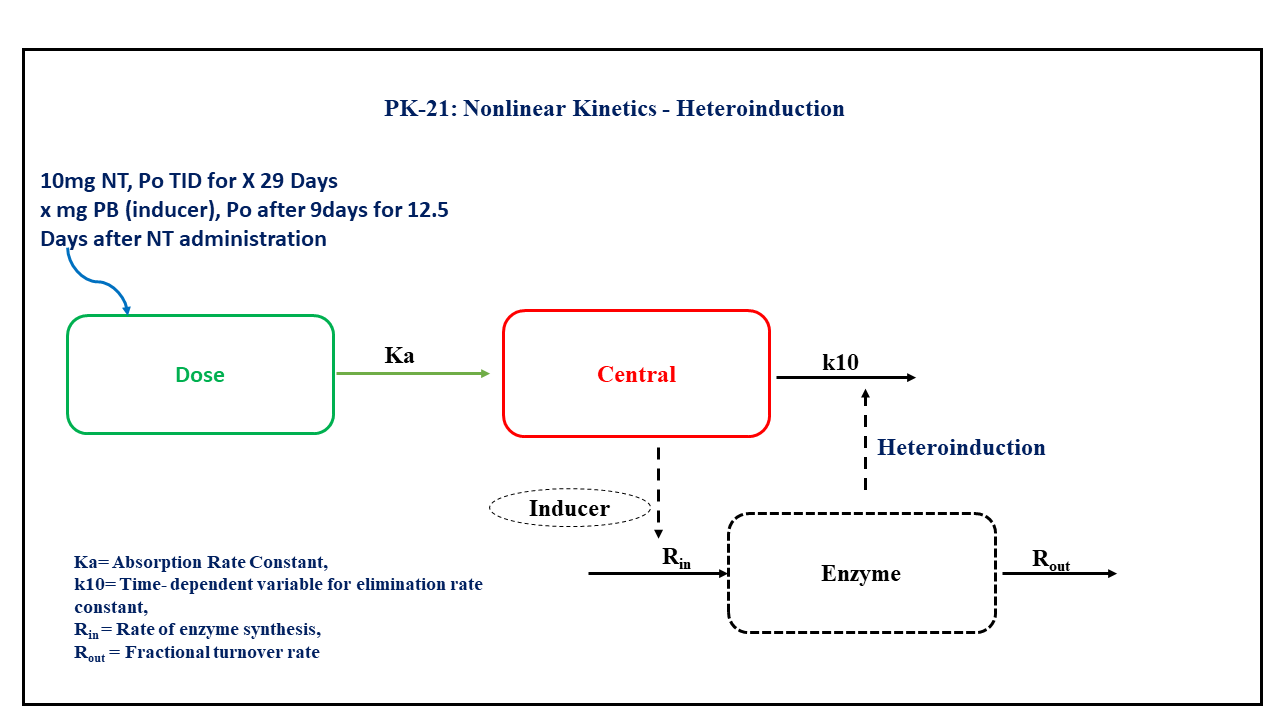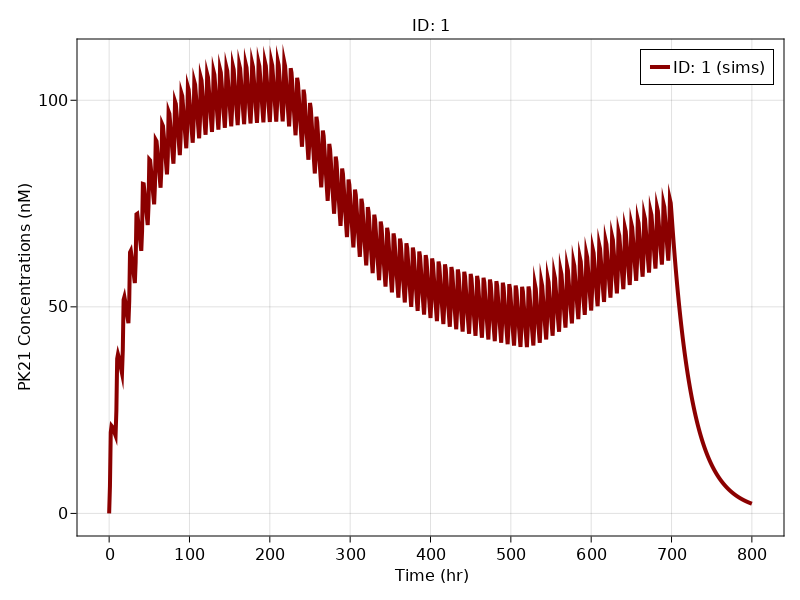# Exercise PK21 - Nonlinear Kinetics - Heteroinduction

### Background

• Structural model - One compartment Oral administration consists of time dependent change in the elimination rate constant.

• Route of administration - Oral

• Dosage Regimen - Nortriptyline (NT) 10 mg (or 10000 μg) Oral, three times daily for 29 days (i.e. 696 hours), after 216 hours treatment with an enzyme inducer i.e. Pentobarbital (PB) for a period of 300 hours i.e up till 516 hours of treatment with NT.

• Number of Subjects - 1### Learning Outcome

We will learn how to simulate from a heteroinduction model after repeated oral dose data on treatment with an enzyme inducer for a limited duration.

### Objectives

In this exercise you will learn how to simulate from an Oral One Compartment model with enzyme induction where clearance is time dependent.

Certain assumptions to be considered:

• The fractional turnover rate i.e. $Kout$ of the enzyme has a longer half-life than the drug or the inducer

• The duration from one level of enzyme activity to other will be influenced by $Kout$ of the enzyme

• The $Kout$ is not regulated by the Pentobarbital. The interpretation $V$ includes bioavailability (i.e., it is really $V/F$).

### Libraries

Call the "necessary" libraries to get start.

using Random
using Pumas
using PumasUtilities
using CairoMakie


### Model

In this one compartment model, we administer dose in Depot compartment at 'time= 0' that is given every '8 hours' for '87 additional doses'. A second drug which is an enzyme inducer (Pentobarbital) is added at 216 hrs for 300 hrs up to 516 hours of treatment with Nortriptyline.

Note:- We do not have concentrations of Pentobarbital and hence it is not included in the model.

pk_21        = @model begin
desc     = "Heteroinduction Model"
timeu    = u"hr"
end

@param begin
"Absorption Rate Constant (1/hr)"
tvka     ∈ RealDomain(lower=0)
"Intrinsic Clearance post-treatment (L/hr)"
tvclss   ∈ RealDomain(lower=0)
"Lag-time (hrs)"
tvlag    ∈ RealDomain(lower=0)
"Intrinsic Clearance pre-treatment (L/hr)"
tvclpre  ∈ RealDomain(lower=0)
"Fractional turnover rate (1/hr)"
tvkout   ∈ RealDomain(lower=0)
"Volume of distribution (L)"
tvv      ∈ RealDomain(lower=0)
Ω        ∈ PDiagDomain(3)
"Proportional RUV"
σ²_prop  ∈ RealDomain(lower=0)
end

@random begin
η        ~ MvNormal(Ω)
end

@covariates TBP TBP2

@pre begin
Ka       = tvka * exp(η)
Clpre    = tvclpre * exp(η)
Clss     = tvclss * exp(η)
Vc       = tvv
Kout     = tvkout
Kpre     = Clpre/Vc
Kss      = Clss/Vc
Kperi    = Kss-(Kss-Kpre)*exp(-Kout*(t-TBP))
A        = Kss - (Kss-Kpre)*exp(-Kout*(TBP2-TBP))
Kpost    = Kpre - (Kpre-A)*exp(-Kout*(t-TBP2))
K10      = (t<TBP) * Kpre + (t>=TBP && t<TBP2) * Kperi + (t>=TBP2) * Kpost
end

@dosecontrol begin
lags     = (Depot=tvlag,)
end

@dynamics begin
Depot'   = -Ka*Depot
Central' =  Ka*Depot - K10*Central
end

@derived begin
cp       = @. (1000/263.384)*Central/Vc
"""
Observed Concentration (nM)
"""
dv       ~ @. Normal(cp, sqrt(cp^2*σ²_prop))
end
end

PumasModel
Parameters: tvka, tvclss, tvlag, tvclpre, tvkout, tvv, Ω, σ²_prop
Random effects: η
Covariates: TBP, TBP2
Dynamical variables: Depot, Central
Derived: cp, dv
Observed: cp, dv


### Parameters

The parameters are as given below. tv represents the typical value for parameters.

• Ka - Absorption Rate Constant (1/hr)

• CLss - Intrinsic Clearance post-treatment (L/hr),

• tlag - Lag-time (hrs),

• CLpre - Intrinsic Clearance pre-treatment (L/hr),

• Kout - Fractional turnover rate (1/hr),

• V - Volume of distribution (L),

• Ω - Between Subject Variability,

• σ - Residual error.

param = ( tvka    = 1.8406,
tvclss  = 114.344,
tvlag   = 0.814121,
tvclpre = 46.296,
tvkout  = 0.00547243,
tvv     = 1679.4,
Ω       = Diagonal([0.0,0.0,0.0]),
σ²_prop = 0.015)

(tvka = 1.8406, tvclss = 114.344, tvlag = 0.814121, tvclpre = 46.296, tvkou
t = 0.00547243, tvv = 1679.4, Ω = [0.0 0.0 0.0; 0.0 0.0 0.0; 0.0 0.0 0.0],
σ²_prop = 0.015)


### Dosage Regimen

In this section the Dosage regimen is mentioned for:

• Oral dosing of 10 mg or 10000 μg at time=0 that is given every 8 hours for 87 additional doses for a single subject.

ev1  = DosageRegimen(10000, cmt = 1, time = 0, ii = 8, addl = 87)
sub1 = Subject(id = 1, events = ev1, covariates =(TBP = 216, TBP2 = 516),
observations = (cp = nothing,))

Subject
ID: 1
Events: 88
Observations: cp: (nothing)
Covariates: TBP, TBP2


### Simulation

Let's simulate for plasma concentration with the specific observation time points after Oral administration of NT before, during and after treatment with PB.

Random.seed!(123)
sim_sub1 = simobs(pk_21, sub1, param, obstimes = 0:1:800)


### Visualization

f, a, p = sim_plot(pk_21, [sim_sub1],
observations = :cp,
color = :redsblues,
linewidth = 4,
axis = (xlabel = "Time (hr)",
ylabel = "PK21 Concentrations (nM)",
xticks = 0:100:800))
axislegend(a)
f